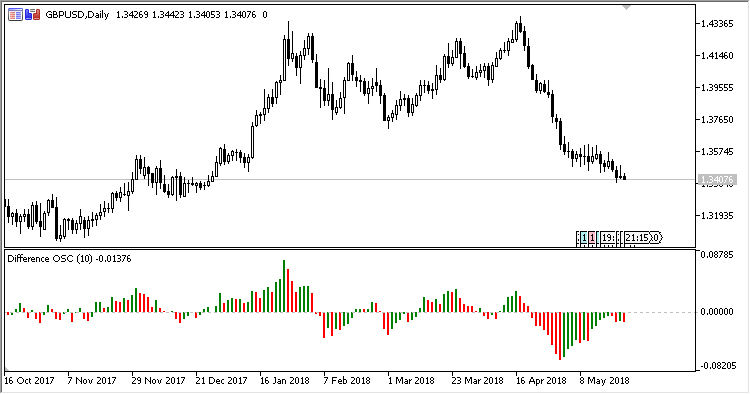# Difference2 – indicator for MetaTrader 5

Indicator Difference2 shows the difference between the current price and the price as of N periods ago, as a histogram.

Unlike the first version of Difference, where the difference is shown between the values of bar prices (High – Low or Close – Open) now and as of N periods ago, this version shows the difference between the Applied prices as of now and as of N periods ago.

It has three input parameters:

• Period – period;
• Calculation type – type of calculations:
• Absolute – calculations in absolute values;
• Relative – calculations in relative values.
• Applied price – price used for calculations.
Related Posts

Calculations:

Calculation type = Absolute:

`Difference[i] = Price[i] - Price[i-Period]`

Calculation type = Relative:

`Difference[i] = 100*(Price[i] - Price[i-Period])/Price[i-Period]`